All submissions of the EM system will be redirected to Online Manuscript Submission System. Authors are requested to submit articles directly to Online Manuscript Submission System of respective journal.

# Review

, Volume: 7( 3) DOI: 10.37532/2320-6756.2019.7(3).182

## New Concept of Physics Energy Behaviour and its Application in Cosmology to Define Gravity Value from Einstein's Relativity

*Correspondence:
Houssam K, LGCE of University of Jijel, Algeria, E-Mail: khelalfahoussam@gmail.com

Received: May 28, 2019; Accepted: June 6, 2019; Published: June 12, 2019

Citation: Houssam K. New Concept of Physics Energy Behaviour and its Application in Cosmology to Define Gravity Value from Einstein's Relativity. J Phys Astron. 2019;7(2):182.

### Abstract

The goal is to define Quantum Gravity Value by combination between our new concept about physics energy behaviour and Einstein relativity's Theory. Our theory is based on the existence of a relationship between energy and vacuum!, So; it can be considered that the energy is a function of the vacuum ratio. Therefore; we can say that vacuum ratio is constitutes a part of space-time. With simple mathematical formula we can easily obtain the equation of the Energy Vacuum. This gives us the distribution of the energy Vacuum (E) into two parts are inversely proportional from our vacuum energy diagram, the effective energy that the sole responsible for Curvature of space-time fabric, and the lost energy that the responsible of the Gravitational waves. From this equations we can find that the relationship with Energy and Vacuum ratio is linear which are compatible with Quantum Mechanics laws and Maintains the energy conservation principle. It is also observed that the equations obtained through our theory are Combining relativity and Quantum Mechanics into one continuum. If we take the equations of our theory, we can easily obtain from Curvature of Space-Time Fabric the Gravity value equation which equal to the square root of energy multiply times the square of the vacuum ratio. On other hand; A curvature matrix and a Time Dilation's Circle are proposed, which gives us a new method to facilitate the calculations of the parameters involved in the Space-Time Curvature.

## Keywords

Energy; Vacuum ratio; Relativity; Quantum mechanics; Quantum gravity; Cosmology; New theory

## Introduction

Energy (E) is one of the obsessions of humanity concerns since antiquity. The feasibility of formulating the Singularity of Nature was enunciated by Einstein's mathematical formula, demonstrating the equivalency of energy and mass (E=m*c2) [1-9]. Despite that statement of principle, it has proven difficult to achieve this goal scientifically by directly merging Relativity and Quantum Mechanics into one continuum [10,11]. It has been realized that there are four forces in the universe; gravity, electromagnetism, weak and powerful nuclear forces. The universe according to Stephen Hawking is Energy, space and time [12,13]. Perhaps there is a fifth force in the universe; the force of the Vacuum represented with the vacuum ratio (v) ?!.

“Gravity is one of the mysteries to be solved in order to get a complete understanding of how the Universe works. So, what is gravity and where does it come from? To be honest, we’re not entirely sure” . In general relativity, the universe has three dimensions of space and one of time and putting them together we get four-dimensional space-time, which gravity as an emergent effect from the space-time curvature associated with distributions of energy. "Matter tells space how to bend; space tells matter how to move" . Despite intense efforts over the last years, it is far from clear at this time what a consistent theory of quantum gravity will look like and what its main features will be . Although many books and articles on quantum gravity and graviton have been published, no explanation has yet been provided. In fact, the old definition of gravity  is not able to solve the quantum gravity problem. It means we need a new definition of gravity that should be based on developing old theories and experimental evidence and resolve the renormalization problem. This research is concerned with the unification of general relativity and quantum mechanics into a theory of quantum gravity value .

New Concept

The relation of the Space-Time Fabric to a Cosmic Energy impact during their interaction is very complex (FIG. 1a). This may be the reason for the slow progress in the development of a rational method for Curvature of Space-Time Fabric analysis. Very few theoretical models are available. From the US; another theory  (FIG. 1b) of the interaction between Space-Time Fabric and Cosmic Energy has been proposed to facilitate analysis of Gravitational Waves results, (FIG. 1c). Our theory is based on the existence of a reflection of a part of the energy received by the matrix of the Space-Time Fabric to be interacted with Cosmic Energy (FIG. 1b), which implies a distribution of energy in two parts (Eq. 1), the effective energy (E '), which is solely responsible for Space-Time Curvature, and the lost energy (EL) responsible for resultant Gravitational Waves:

FIG. 1. (a): Curvature of Space-Time Fabric after, Einstein ; (b): New concept of energy behavior interacted to Space-Time Fabric after, H. Khelalfa ; (c) Gravitational waves .(1)

Space-time and energy interaction mechanism: Our theory is based to consider the space-time fabric as element volume of a fluid [11,19] or Continuous media which give us the possibility to applied Continuous mechanical environments laws (FIG. 2) characterized by a vacuum ratio (v). So; The physical Curvature (ΔV) of the Space-Time Fabric caused by Energy pulses (E) will reduce the vacuum ratio (v) by filling the vacuum with energy. Therefore, it can be considered that the energy is a function of the vacuum ratio (e) Eq. 2:

Fig. 2. Definition and components of stress of space-time fabric's element volume according to our theory.(2)

When Space-Time Fabric extend in the unknown matter (or dark matter), a high proportion of the Energy impulse is transferred to the porous unknown matter. In this case we will consider that the energy is a function of degree of saturation of unknown matter (S) instead of the vacuum ratio Eq. 3:(3)

Case of space-time fabric without unknown matter: Without unknown matter, the physical displacement of the Space-Time skeleton (pothole) is the main mechanism of Curvature. During the displacement process (FIG. 3), high energy waves propagate through the Space-Time Fabric, Thereby, each Space-Time Fabric element experiences strong impulsion energy for a period of time, resulting from the filling of constituent vacuum ratio in the volume in which they are reduced. Given the Eq. 2, we deduce the Eq. 4:

FIG. 3. Overall mechanism of Space-Time Fabric and Energy interaction in Case without unknown matter .(4)

On the other hand, it is also known that damping (amortization ) occurs at infilling of vacuum by energy, which manifests itself as an decrease in the vacuum ratio as a function of time. when the damping (attenuation) in the system exceeds a critical value (v = 0%), there is a starting in the Gravitational waves as a function of time. Given the Eq. 2, we deduce the Eq. 5:(5)

Case of space-time fabric with unknown (or dark) matter: Space-Time Fabric interaction to Cosmic Energy in saturated Space-Time Fabric with unknown (or dark) matter is different and of course more complex (FIG. 4). Its applicability in a saturated vacuum is generally considered less effective due to the fact that some of the applied energy is absorbed by interstitial matter (vacuum matter). considering the Eq. 3, we deduce the Eq. 6:

FIG. 4. The overall mechanism of Space-Time Fabric and Energy interaction in Case with unknown matter .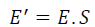(6)

Moderate levels of energy impulse could cause the displacement of previously vacuum areas in the Space-Time Fabric without unknown matter, Saturation means that these cavities are filled with vacuum matter and that the displacement would be inhibited by unknown matter (vacuum matter) resistance to curvature. However, the really saturated Space-Time Fabric may have a few percents of the total vacuum non-occupied by unknown matter. In this condition, the intensity of the Gravitational Waves would be greatly increased due to the low bulk of vacuum matter, but the impact energy still ensures the curvature of the Space-Time Fabric due to the reduction of the rest vacuum volume. Given the Eq. 3 we deduce the Eq. 7:(7)

As mentioned above, the curvature of the rest vacuum volume has a significant effect on the behavior of saturated Space-Time Fabric with the unknown matter under energy impact. It is accepted that unknown matter contains tiny vacuum pockets enclosed in vacuum volume. An accurate assessment of the volume of vacuum pockets trapped in the unknown matter is difficult to affect the curvature (displacement) of vacuum-matter mixtures and thus significantly alter the process of generating vacuum matter-energy. It is therefore essential to take into account the curvature of the vacuum -matter mixture, instead of the curvature of pure matter. Similarly, based on the Eq.3, determining the energy of the vacuum -matter mixture is as follows (Eq. 8):(8)

Where; Eam is the energy of the vacuum -matter mixture, Ea is the energy of the vacuum, therefore the lost energy is the energy of the vacuum (Eq. 9):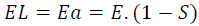(9)

Energy Vacuum Diagram

If we take all the equations from 1 to 9 above we can easily obtain the Energy Vacuum Diagram (FIG. 5); which is clearly illustrated; that the effective energy is proportional to the degree of saturation and the vacuum ratio as opposed to the lost energy. It can be concluded that the Space-Time Fabric response to the cosmic Energy Interaction depends on the vacuum ratio and degree of saturation. Thus, the level of curvature efficiency depends on the effective energy, too, the lost energy is minimal in saturated Space-Time Fabric with unknown matter (or dark/others matter).

FIG. 5. Energy Vacuum Diagram of Interaction of Cosmic Energy and Space-Time Fabric (a); without unknown matter; (b): with unknown matter .

Gravitational Waves Intensity

In Space-Time Fabric without unknown matter, the graph of the Gravitational Waves Intensity is divided into four parts (Very Strong, Strong, Low and Limited and Low Gravitational Waves Intensity) as shown in FIG. 6a to give us an approach to estimate the process of Interaction between cosmic Energy and Space-Time Fabric and its effect (Gravitational Waves). In saturated Space-Time Fabric with unknown (or dark) matter; Assuming the amount of vacuum varies at most between 15 to 25%; which implies a degree of saturation between 75 and at least 85% (FIG. 6b), which limits the applicable interaction field of Cosmic Energy in saturated Space-Time Fabric, and we can consider the rest as a field not practically interacted. Consequently; The most important remark is that the lost energy in the applicable field is very big.

FIG. 6. Graph of Gravitational Waves Intensity of Interaction of Cosmic Energy and Space-Time Fabric (a): without unknown matter; (b): with unknown matter .

Gravity value from space-time curvature

We proposed a relationship to predict the Space-Time Fabric Curvature is as follows :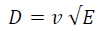(10)

Where; D is the Interacted depth of curvature of Space-Time Fabric (Z=∞), E (%) is the Cosmic Energy to interact, and v (from 0 to 100% or 0 to 1) is vacuum ratio that is fundamentally characterized the Space-Time Fabric volume.

If we apply our theory in Space-Time Fabric without unknown matter, given Eq. 10, we get Eq. 11 :(11)

If we apply our theory in saturated Space-Time Fabric with the unknown matter, given Eq. 10, we get Eq. 12 :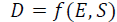(12)

Similarly, for the previous equation, we propose the following equality :(13)

When finishes Interaction between a Space-Time Fabric's element volume and Energy, finding a crater which represents Space-Time Curvature (or Gravity from Einstein Relativity) (FIG. 7) whose volume (ΔV) can be defined by Eq.14 :(14)

Where; V is the total volume of the Space-Time Fabric's element, A is the surface/or area of the Interacted Energy, ΔH is the height of the crater (Space-Time Curvature) and D is the Interacted depth of curvature of Space-Time Fabric mentioned in Eq. 10 above.

After the development of Eq. 14 and introducing Eq. 11 we deduce Eq. 15 :

Which implies the equation Eq. 16.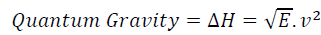(16)

We found an empirical relationship between the height of the crater (Space-Time Curvature) and interacted depth determined by Eq. 17, where we introduce the proposed coefficient K (TABLE 1) :

 v v<0.3 0.30.8 x 2 3 5 7

TABLE 1. The values of the coefficient ?x? according to the vacuum ratio.(17)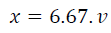(18)

Space-Time Curvature Matrix

As illustrated in FIG. 8 according to our study; the Space-Time Fabric curvature phenomenon has been summarized in a curvature cycle which consists of three basic components, Energy (E), times (T) and Curvature (C), that they are related to each other by three main index properties, over curvature ratio (OCR), Curvature index (Cc) and anti-gravity index (Cg).

FIG. 8. The Space-Time Fabric Curvature Cycle and its representation on the Cartesian coordinate system according to our theory.

If we take all assuming that indexes and components are linearly dependent we can easily obtain a curvature matrix, which clearly illustrates that three basic components are diagonal and the other three main index properties compose the rest of the matrix:Curvature Matrix

Nature of Space-Time Fabric typically displays a complex concept as a result of missing theory about its interaction with Cosmic Energy. A key aspect for the selection of representative Space-Time Fabric parameters is to consider Knowledge of its properties. This nature is based on our theory to determine this characteristic (curvature index Cc, Anti-gravity index Cg, Vacuum ratio v, Over curvature ratio OCR) of the Space-Time Fabric; by using these curvature parameters, it is possible to determine the Curvature (gravity), Times dilation, and amount of interacted Energ, etc. While the rigorous selection of Space-Time Fabric parameters requires a deep understanding and proper knowledge of its behavior. Parameters such as OCR, Cc and Cg play a key role on Curvature and Interacted Energy predictions.

Curvature index (Cc) and Anti-gravity index (Cg) obtained from our theory are necessary for the calculation for Space-Time Fabric curvature. In our hypothesis, we have been looking for a possible relationship between Cc and Cg indices and general characteristics of Space-Time Fabric. One of these parameters is over curvature ratio (OCR). In this study, the effect of OCR and vacuum ratio (v) on Cc and Cg indices, was proposed (FIG. 9-13). Thus, The hypothesis indicates that Cc and Cg indices were influenced by OCR and v, and, a linear relationship between them should be observed. Increasing values of (v) will decrease Cc and Cg values. On the contrary, when OCR increases, Cc and Cg values would also increase. It is possible to say that the Cc and Cg values are influenced by the same parameters. Therefore it can be concluded that the amount of Curvature depends on the Anti-gravity index and that the amount of Curvature (C) is proportional to the Anti-gravity index (Cg) (FIG. 14 and TABLE 2). When we compare the time dilation required to stabilize the Curvature, we can talk about OCR and Cc. Therefore it can be concluded that the time dilation depends on the Curvature index (Cc) and over consolidation ratio (OCR) (FIG. 15 and TABLE 2) and that the times of Curvature (T) is proportional to the Curvature index (Cc) and over consolidation ratio (OCR), and at the same time that the two latter are proportional.

FIG. 14. Correlation should be; between Amount of curvature (C) Vs. Anti-Gravity index (Cg) according to our hypothesis.

FIG. 15. Correlation should be; between required time dilation to stabilize space-time fabric curvature vs. OCR vs. CC according to our hypothesis.

Parameter Curvature Vacuum ratio,
v (%)
Energy,
E (%)
Curvature Index,
Cc
Anti-gravity Index, Cg Over curvature ratio,
OCR
Equation 0 ~ ∞ (infinity is a black hole) 0 ~ 100 =E' + EL =0.510(v - 0.33) =0.15(v +0.007) =E'/ EL

TABLE 2. Correlation parameters of space-time fabric curvature according to our hypothesis.

Main Parameters Responsible for Space-Time Fabric Behavior and Time Dilation (Td)

Our theory introduced the following factors as the main causes of Time Dilation (Td) according to Space-Time Fabric Curvature (FIG. 16) (Eq. 19, Eq. 20): over curvature ration (OCR), Curvature (C), and phase (Phi) (or time evolution) which depends the Curvature index (Cc) as a function of (t) and Anti-gravity index (Cg) as a function of (X). It means that that the change in the value C involves changing the value of Td.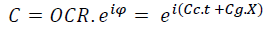(19)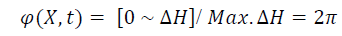(20)

In order to carry more than one value and indices to represent the Time Dilation Circle, We used the complex number to explain the curvature characteristics of Space-Time Fabric. We can simply conclude that the final state equals the initial state multiply by the time dilation (Td) (Eq. 21).(21)

The most determining factor for the credibility Time Dilation's Circle is its ability to Contain all the characteristics and indices contributing to this phenomenon as illustrates (FIG. 16, Eq. 19).

## Conclusion

Is not there yet a complete theory linking Einstein's relativity to quantum mechanics ?!. Our theory is based on the existence of a relationship between energy and vacuum!, So; it can be considered that the energy is a function of the vacuum ratio E=f (v). With a simple mathematical formula, we can easily obtain the equation of the Energy Vacuum E= E*v+E*(1- v). This gives us the distribution of the energy Vacuum (E) into two parts are inversely proportional from our vacuum energy diagram , the effective energy (E'=E*v) that the sole responsible for Curvature of space-time fabric, and the lost energy (EL=E*(1- v)) that the responsible of the Gravitational waves. Therefore; we can say that vacuum ratio which has a value from 0% to 100% (or from 0 to 1) constitutes a part of space-time. From these equations, we can find that the relationship with Energy and Vacuum ratio is linear which are compatible with Quantum Mechanics laws and Maintains the energy conservation principle. It is also observed that the equations obtained through our theory are Combining relativity and Quantum Mechanics into one continuum. If we take the equations of our theory, we can easily obtain from Curvature of Space-Time Fabric the Quantum Gravity value equation which equals to the square root of energy multiple times the square of the vacuum ratio (Quantum Gravity=√E*v^2). From the consequences, we have found after our theory that gravity is energy? On another hand; our theory consists in considering Space-Time as element volume of a fluid or Continuous media, which give us the possibility for using Finite Element Method (FEM) to resolve and simulate Space-Time Fabric Curvature according to Navier-Stokes equations. Energy Vacuum Diagram as theory can be given new and good explanations in physics about cosmic, quantum and relativity phenomenon it will make laboratory experiments or cosmic monitoring.

The Interacted Energy consists of displacing Space-Time Fabric and increasing the time dilation. The effectiveness of this hypothesis was demonstrated by the results of the available propositions which verified by the curvature matrix. In addition, this matrix gives us a new method to facilitate the calculations of the parameters involved in the Space-Time Curvature, which gives great credibility to our hypothesis. Maybe our hypothesis about the relationship between Indices involved in curvature cycle based on theoretical results caused by lack of equipment, but can be proved in future by experimental tests in order to compare them by our theory. Indeed, Time Dilation's Circle is considered a good new tool to calculate, interpret and explain this phenomenon; moreover, maybe it can clarify us more another cosmological phenomenon in the future?

## Acknowledgment

This work was supported and funded by the K.E.C Laboratory, Department of Engineering and Applied Science of K.E.C Laboratory, Jijel, Algeria, under the project number (PN): KEC.LAB.DEAS.ASTPHY.NCEERQGVP2018N007.

I would like to thank the researchers Dr. Maher Sawaf and Pr. Nidhal Guessoum for his researches and explanation lessons.

### References

1. Houssam Khelalfa, New Concept of physics energy behavior. 2017.
2. Houssam Khelalfa (2019) New theory of soil response to a high energy impact and its environmental consideration. In: Ameen H., Sorour T. (eds) Sustainability Issues in Environmental Geotechnics. GeoMEast 2018.
3. https://www.researchgate.net/publication/330500640_New_Concept_of_physics_energy_behaviour_and_its_application_in_cosmology_to_define_Gravity_value_from_Einstein's_relativity
4. Einstein, Albert. Relativity: The special and general theory. 1920: Henry Holt and Company.
5. Amber L Stuver, Gravitational Waves. 2019.
6. Torday JS. The Singularity of nature, Progress in Biophysics and Molecular Biology. 2019;142:23-31.
7. http://www.alberteinsteinsite.com
8. https://archive.org/details/berdiespezielle00unkngoog/page/n4
9. Rainer Dick. Special and general relativity; An introduction to space-time and gravitation. 2019.
10. Martinetti P. Emergence of time in quantum gravity: is time necessarily flowing? KronoScope. 2013;13:67-84.
11. Yurij VB. Foundation of relativistic astrophysics: Curvature of Riemannian space versus relativistic quantum field in Minkowski space. 2017.
12. Hawking SW, Ellis GFR. The large-scale structure of space-time. 1973.
13. Minkowski H. Space and time. The principle of relativity.1952:75-91.
14. https://www.universetoday.com/75705/where-does-gravity-come-from/
15. https://web.archive.org/web/20120206225139/http:/www.alberteinstein.info/gallery/gtext3.html
16. Hermann N. Quantum gravity and unified theories.
18. Piaggio HTH. Einstein A, " The concept of space”, Nature. 1930;125:897-98.
19. T Padmanabhan. The hydrodynamics of atoms of space-time: Gravitational field equation is navier-stockes equation. 2011;20:2817-22.
Welcome Message

###### Citations : 291

Journal of Physics & Astronomy received 291 citations as per Google Scholar report

#### Indexed In

• Open J Gate
• China National Knowledge Infrastructure (CNKI)
• Cosmos IF
• Directory of Research Journal Indexing (DRJI)
• MIAR
• Secret Search Engine Labs
• Euro Pub
• clarivate-web-of-science-logo-vector
• ICMJE

View More

Berlin, Germany

### World Congress on Quantum Physics

Berlin, Germany